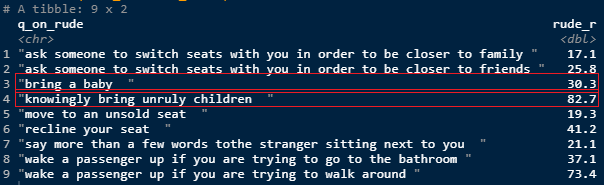top of page
Search
• ♫Shokolatte♬

# Today's Visualization (R)code (complete):

```flying_etiquette <- read.csv("https://raw.githubusercontent.com/ShokoLocoMocco/data/master/flying-etiquette-survey/flying-etiquette.csv")

flying_etiquette_f <- flying_etiquette %>%
mutate_if(is.character, as.factor) %>%
filter("How.often.do.you.travel.by.plane." != "Never")

gathered_data <- flying_etiquette_f %>%
select(contains("rude")) %>%
gather(q_on_rude, res)

dichotimized_fe <- gathered_data %>%
mutate(q_on_rude = str_remove(q_on_rude, ".*rude.to.")) %>%
mutate(q_on_rude = str_remove(q_on_rude, "on.a.plane")) %>%
filter(str_detect(res, ".")) %>%
mutate(rude = if_else(res %in% c('No, not rude at all', 'No, not at all rude'), 0, 1))

rude_behave <- dichotimized_fe %>%
mutate(q_on_rude = gsub("[.]", " ", q_on_rude)) %>%
group_by(q_on_rude) %>%
summarize(rude_r = mean(rude))
rude_behave

initial_bar <- rude_behave %>%
mutate(q_on_rude = fct_reorder(q_on_rude, rude_r)) %>%
ggplot(aes(x = q_on_rude, y = rude_r)) +
geom_col()

initial_bar

bar_labs <- initial_bar +
labs(title = "Hell Is Other People In A Pressurized Metal Tube",
subtitle = "Percentage of 874 air-passenger respondents who said action is very or somewhat rude",
caption = "Source: SurveyMonkey Audience", x = NULL,
y = NULL)

bar_labs

hor_plot <- bar_labs +
coord_flip() +
theme(axis.ticks.x = element_blank(), axis.text.x = element_blank())

hor_plot

end_plot <- hor_plot +
geom_text(aes(label = scales::percent(rude_r), y = rude_r + .05), position = position_dodge(0.9), vjust = 1) +
geom_col(fill = "purple")

end_plot

```

findings:

```rude_behave %>%
mutate(rude_r = rude_r*100)```

👇I never like it when the world is not nice to kids and (mostly) mothers are to blame in such situation.... I also understand there are moments that we don't want to be distracted. I hope this survey would help air companies to come up with some sort of solution for traveling kids so everyone can fly in peace.

*Btw, I wouldn't mind adults be just kicked out for not wearing a mask properly. They just need to act their age instead of acting unruly knowingly themselves.